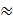Sample ProblemThree people are pulling on your right arm.

A force with magnitude 100 pounds pulls on your arm (at angle 60° from positive x-axis).

Another force with magnitude 120 pounds pulls on your arm

(at angle -30° from the positive x-axis).

A final force with magnitude 150 pounds pulls on your arm (at angle 270° from positive x-axis).

Find the direction and magnitude of the resultant of these forces.

Direction of resultant force: ° (round to one decimal place)

Magnitude of resultant force: pounds (round to one decimal place)

Solution

F1=100<cos 60°, sin 60°>=<50, 50√3>

F2=120<cos -30°, sin -30°>=<60√3, -60>

F3=150<cos 270°, sin 270°>=<0, -150>

Fresulting=<50+60√3+0, 50√3-60-150>=<50+60√3, 50√3-210>

||Fresulting||=197.3 lbs

tan(50√3-210/50+60√3)=θ

θ-38.7°